# Conformal radius of a domain

(diff) ← Older revision | Latest revision (diff) | Newer revision → (diff)

A characteristic of a conformal mapping of a simply-connected domain, defined as follows: Letbe a simply-connected domain with more than one boundary point in the-plane. Let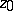be a point of. If, then there exists a unique function, holomorphic in, normalized by the conditions,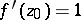, that mapsunivalently onto the disc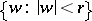. The radiusof this disc is called the conformal radius ofrelative to. If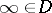, then there exists a unique function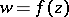, holomorphic inexcept at, that, in a neighbourhood of, has a Laurent expansion of the formand that mapsunivalently onto a domain. In this case the quantity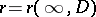is called the conformal radius ofrelative to infinity. The conformal radius of,, relative to infinity is equal to the transfinite diameter of the boundaryofand to the capacity of the set.

An extension of the notion of the conformal radius of a domain to the case of an arbitrary domainin the complex-plane is that of the interior radius ofrelative to a point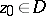(in the non-Soviet literature the term "interior radius" is used primarily in the case of a simply-connected domain). Letbe a domain in the complex-plane, letbe a point ofand suppose that a Green function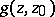forwith pole atexists. Letbe the Robin constant ofwith respect to, i.e.The quantity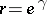is called the interior radius ofrelative to. Ifis a simply-connected domain whose boundary contains at least two points, then the interior radius ofrelative tois equal to the conformal radius ofrelative to. The interior radius of a domain is non-decreasing as the domain increases: If the domains,have Green functions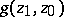,, respectively, ifand if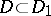, then the following inequality holds for their interior radii,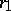at: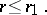The interior radius of an arbitrary domainrelative to a pointis defined as the least upper bound of the set of interior radii atof all domains containing, contained inand having a Green function. In accordance with this definition, ifdoes not have a generalized Green function, then the interior radiusofatis equal to.

How to Cite This Entry:
Conformal radius of a domain. G.V. Kuz'mina (originator), Encyclopedia of Mathematics. URL: http://encyclopediaofmath.org/index.php?title=Conformal_radius_of_a_domain&oldid=18740
This text originally appeared in Encyclopedia of Mathematics - ISBN 1402006098